# The formula of vergence

The formula of vergence is very useful in ophthalmology. It is used to calculate the position which forms the image of an object after refraction of light through an optical system: this system can be simple (a thin lens) or complex (the couple cornea (+ lens). The vergence - or optical power paraxial - expressed in diopters. It is equal to theinverse of the distance which separates it from the top of the optical system of the plan or form the net image (specifically plan main image to the image in optics paraxial focus).

The use of the formula of vergence to do various calculations without needing to calculate the path of many rays through the optical system. It allows to establish a simple formula for the calculation of the power of the implant asked during the cataract surgery. It also allows to establish the formulas to convert the power of a telescope from a power of lens glass (and vice versa) according to the glass eye distance.

The vergence of the eye comes from the sum of the vergence of the cornea and of the vergence of the lens. In the accommodation, the vergence of the lens increases (the lens distorts and adopts a more significant curvature).

The formula of vergence is the position of the source object, its distance with the optical system, and the vergence, or optical power of this system.

This page is dedicated to the formula of vergence: its origin is reported, and its some applications mentioned.

## Origin of the formula of vergence

Vergence can be established from the law establishing the route after refraction of light rays: law of Snell (or Snell-Desmaps) applied in paraxiales conditions. It stems from some approximations that can be done in these circumstances, where we consider the part of the optical system close to the optical axis, (paraxiales conditions) and where the rays form a low angle with this axis. Vergence formula to calculate the position of the image from a source without needing to use a calculation of ray-tracing (ray tracing).

An example of a simple optical system, and an object source (S) located very far from it, to infinity: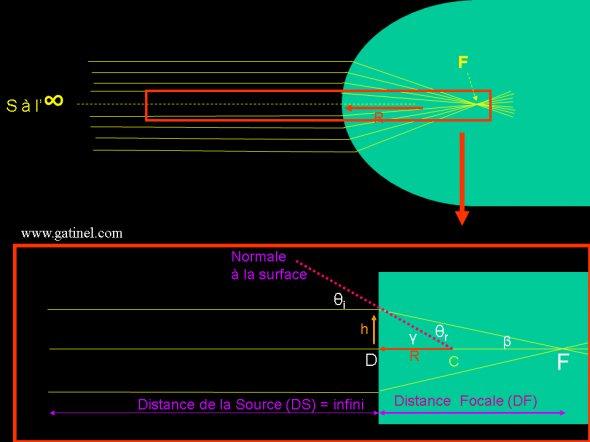An optical system in the paraxiales conditions can be approached as 'flat' in the region very close to the optical axis. This allows to achieve the geometric approximations useful for the calculation of the path of the rays refracted by the system. In the present case, the rays are parallel to the optical axis, and in the paraxiales conditions, we will see that these rays are focused toward the fireplace of the system (which should not be confused with the center of curvature C). Θi and θt are the angles of incidence and transmission of the considered Ray: they are defined in terms of the 'normal' at the point of impact. In the diagram, because the approximation made (the surface is flat), normal is not perpendicular to the surface: for small angles, the difference is negligible.

Consider a general case, where the source is located not at infinity, but at a distance O, and bathes in a middle of index n. The optical system is a spherical diopter index and RADIUS R is. We know n, no, (the distance from the source to the dioptre) O and R, the radius of the top of the dioptre.

The question that can solve the vergence formula is: where is formed the image I ?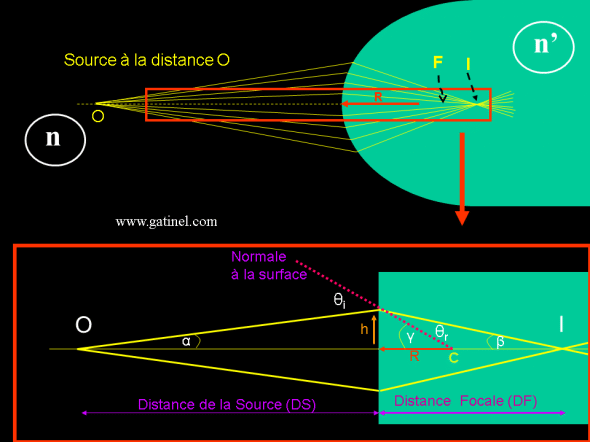The source is now placed at a finite distance O. In the paraxiales conditions, the rays are refracted and intersect in a point in I, who is the image of point O. I is located at the back of F, which is the focal length of the system (but we don't need at this point): Snell's law is enough !).

Snell's law allows to write: n sin θi = no sin θt

In paraxiales conditions, we can replace sin θi by θi in radians value. The equation becomes:

n θi = no θt

We must now express θi and sin θt terms easier to measure variables: (remember that we know O, R and).

Looking at the figure, you can ask: θi = α + γ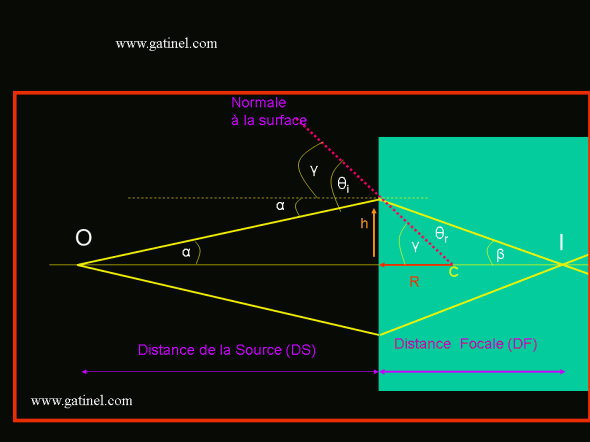The following figure gives the angle of incidence, θi as the sum of the angle of the RADIUS with the optical axis α and the angle between the segment linking the entry point and the center of curvature and the optical axis γ.

Similarly, it is possible to verify that θt = γ - β

We can then replace θi = α + γ and θi = α + γ in Snell's law to get:

n (α + γ) = No (γ - β)

n α + n γ = no γ - no β

n no β = γ + α (is - is)

One can express the angles α, γ and β based on h, O, i, and R (recall that we know O and R values).

In the paraxiales (for small angles) conditions:

Tan α = α = h/o = - h/O (O distance is negative by convention; the distance is measured to the left)

Tan γ = γ = h/R

Tan β = β = h/I

At this point, just to know: to find the value of I. However, we do not know this value. However, if we replace the values of the angles by these reports we get:

n no β = γ + α (not - only)-> n h/O + h/I = h (no - n) / r

We recognize the paraxial formula : nu + don't u' = h (no-n) / r by asking u = h/O and u'= h/I. To get these two equalities, it took to make a paraxial on small angle approximation.

We can simplify the formula by h and one obtains finally:

n/O + No /I = (no - n) / R

either

No /I = n/O + (no - n) / r

Can then be calculated I, since is "dumped" the unknowns (angles, and height of incidence h).

We appreciate the power of this formula, which requires to know the distance of the source with the dioptre object O (which we know curvature R and index don't) to simply calculate the distance the image I!

The term 'vergence' comes from the fact that each term is the opposite of a distance: (no - n) / R is the vergence, or refractive power, of the dioptre. The inverse of a distance expressed in diopters (recall that n and are not dimensionless, since they correspond to the ratio of the speed of light in a vacuum and the speed of light in the middle).

For example, if the diopter has a hint of 1.37, and a radius of curvature R of 8 mm, the vergence of the diopter in the air is equal to (1.33 - 1) / 0.008 is 41.25 diopters.

n/O is the vergence of the Vo object

No /I is the vergence of the Vi image

and (not - n) /R is the optical power P of the dioptre.

Thus, one can write: Vo + P = Vi

To calculate these vergences, take into account the sign of the distances: negative to the left, positive to the right.

## Applications - examples

### Magnifying glass

Without a schema, this formula allows to simply calculate the place or forming the image of an object looked through a magnifying glass. If the Magnifier to a power of 25 D, and one approach to 2 cm of the object (for example a stamp) we can ask that:

the vergence of the stamp plus the vergence of the Magnifier is the vergence of the image.

The vergence of the stamp is 1 /-0.02 (in meters) = - 50 D the sign is negative if the magnifying glass is to the right of the stamp. The vergence of the Magnifier is 25 D. The vergence of the image is equal to-50 + 25 = - 25 D. To get the vergence of the image, it calculates 1 /-25 =-0.04 metres = - 4 cm. The image is also on the left (on the same side as the stamp).

To calculate the magnification (magnification: M), we can use the formula: M = n x image distance / n x distance object: (here n = 1). The magnification is equal to the image/object distance: the image appears as a stamp twice bigger and located 4 cm behind the magnifying glass.

### Distance glass - eye

The glass eye distance is a parameter that it is important to know, especially for conversion between correction (glasses) and correction in contact lenses.

The refraction of one eye is often expressed as the power of the bezel glass used to correct it. For example, a myopia of-3 D corresponds to a defective vision which is corrected by the installation of a glass-3D. Installation of this glass is performed on a test or in a refractor auto mount: the glass is located at a distance of the eye: about 12-15 mm.

The distance from the glass with the image of the eye focus is not equal that of the plan main image of the eye with this image focus. Yet, the 'real' eye refraction must be calculated as the inverse of the distance between the image plane and the image focus, and not between the distance of the corrector glass and the image focus.

We can use the vergence formulas to predict the difference between 'real' eye refraction and ocular refraction expressed in the plan of glasses. This difference, if material, will be interest to convert refraction refraction 'lenses' "glasses": indeed, one can consider that the corneal plan (where the lens is placed) is confused with the plan main image of the eye (actually located 1.5 mm behind the cornea).

The following diagram represents a farsighted eye corrected by a glass of power (in dioptres).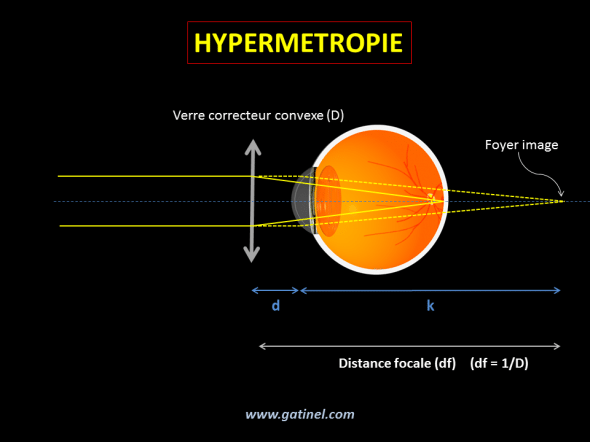A farsighted corrected by a convex glass of positive power (ex: + 5 D). The effect of the glass is to compensate for the flaw of vergence is reached the hyperopic eye. When the glass is positioned in front of the eye of the patient at a distance d, the rays from a distant source are focused in the retina, and either at the back of this plan. The purpose of glass fireplace is located at home eye uncorrected image. The image focus is located at the distance the plan k main image of the eye (compared here to that of the cornea).

The vergence of the eye is called K (in diopters): it is equal to 1/k (k is a distance in meters). the focal length of the glass is called df.

We can put k = df - d, where K = 1 /(df – d). Using the equation D = 1/df we get:

K = D /(1 – d x D) : this formula is used to calculate the refraction of the eye (or a contact lens power) from the power of the corrector (D) glass and glass-eye (d) distance.  When d = 0, K = D

Knowing that df = K + d, we get:

D = K (1 + d x K) : this formula is used to calculate the refraction of the glass of bezel to ask at a distance d when we know the ocular refraction (or the power of the corrective contact lens).

Examples:

1) A hyperopic eye is corrected by a lens of + 5 D. What is the power of glass providing an equivalent correction, knowing that the glass is placed 13 mm from the eye?

K = 5 d, where k = 1/5 = 0.2 meter

The focal length of the glass must be 0,2 + 0.013 = 0,213 meters.

The power of the glass is equal to 1/0,213 = + 4.70 D.

The vergence of the bezel glass is less than that of the lens.

2) A strong myopic eye is corrected by a-D: 15 bezel glass this glass is placed on a mount located 14 mm from the eye. If the patient wishes a correction in contact lens, what will be the power of it?

The focal length of the glass is 1 / meter - 15 = - 0,0667; so the point of the myopic eye punctum is located at 0,00667 + 0.014 = 0,08067 meters. The lens should focus on: 1/0,08067 =-12,40 diopters.

The difference of vergence between the glass and the lens is important:-2,50 diopters about! More one eye is nearsighted, and the greater the difference of vergence between the glass and the lens. The lens is less powerful than the glass bezel.

In refractive surgery, refraction used to program the laser excimer)LASIK(, PKR) is that of the correction in glasses! When entering this correction, a conversion is performed to calculate the effective correction which is issued in the corneal plan.

### Apparent size of the pupil of the iris

This problem is more complex... pupil irisne has an anatomical diameter (diameter physical that you would measure inside the eye), which is not equal to its apparent diameter: indeed, when we observe the pupil, collected his virtual image seemingly located in front of the anatomical pupil, and whose diameter is greater than the physical diameter : this is the to the "magnification" (magnification) of the image of the pupil irisne by the corneal dome!

This virtual image formed by the cornea is the pupil of entry of the eye. Here, a pattern emerged: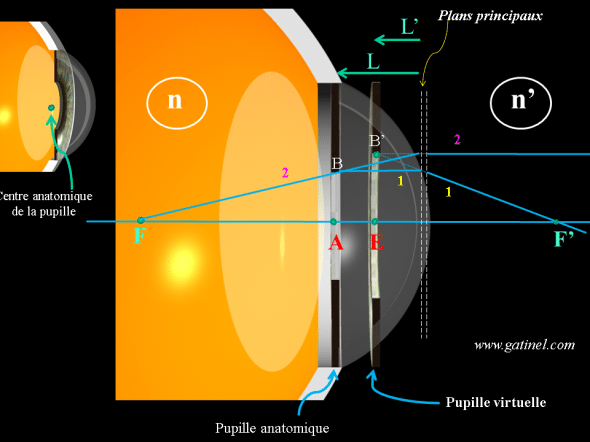The radius of the pupil virtual image is EB', from the anatomical pupil is AB. To calculate the apparent position of the pupil (position of the plan linking point E to point B', corresponding to the distance the on the diagram) we assimilate the cornea to a system in which the cardinal points F and F' (homes) and the major plans are known. For a graphical representation of the position of B', we can draw a RADIUS (1) that seems to come from infinity (from the left) and goes through B parallel to the optical axis of the cornea: this Ray is by definition refracted towards the home F'. A second RADIUS is traced from the F: home it emerges by definition of the cornea that is parallel to the optical axis. The position of B' is the intersection of the extension of the rays 1 and 2 (dotted).

To calculate the position of the 'virtual' pupil plan, we can use the formula of vergence:

Vergence of the cornea + vergence of the object (the pupil) = vergence of the image formed, either:

Vergence of the cornea + n/L = no/l '

We assimilate the cornea to a spherical diopter index (k) is close to that of water (n = 1.33) mood. The vergence of the cornea is the result of the vergence of the anterior and the posterior side: she is close to average 43 dioptres.

n is the refractive index of aqueous (1.33) mood. L is the depth of the anterior chamber (3.5 mm is 0.0035 m) about (directed to the left, L has a negative value)

The refractive index of air is 1. The value of the position of the virtual pupil is equal to: 1 x (-0.0035) / (1.33 - 0.0035 x 43) = - 2.97 mm or 500 microns in front of the anatomic pupil plan.

To calculate the magnification (magnification: M), we can use the formula: M = n x the / no x L = 1.33 x 2.97 / 1 x 3.5 = 1.12: the apparent diameter of the pupil of entry is about 1.12 times larger than that of the anatomical pupil. Conversely, for an apparent diameter of 6 mm, the actual diameter of the pupil is 6/1.12 = 5.35 mm.

The main parameters that affect this calculation are the power of the corneal diopter: the magnification is proportional to the corneal vergence. After corneal refractive surgery for myopia: the vergence is reduced, which can in theory cause a slight reduction in the apparent diameter of the pupil. What precisely about the diameter of the optical zone to program for the correction of myopia, consider the diameter of the pupil of entry (i.e., the apparent diameter), as well as the depth of the anterior Chamber. Other factors are likely to play a role although minimal: the asphericity of the cornea, as well as the optical distortion 'barrel' induced by the cornea (which tends to induce a reduction in the apparent diameter of the pupil).

Finally, the vergence formula allows (and especially) to establish the formula 'mother' theoretical calculation of cataract surgery implantwhich is the subject to a specific page on the site is accessible directly by the link